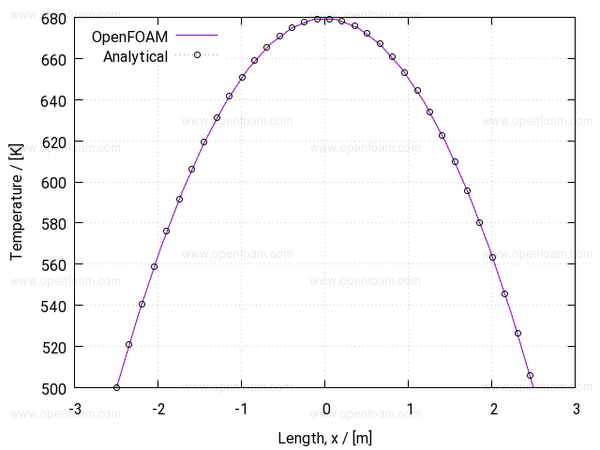This source provides users with the ability to add Joule Heating contributions to any thermal solver.

## Properties🔗

The option solves an equation for the electrical potential, V, of the form

$\div (\sigma \grad V) = 0$

Where $$\sigma$$ (sigma) is the electrical conductivity. The thermal source is then given by

$\dot{Q} = (\sigma \grad V ) \dprod \grad V$

A sample result is shown below## Usage🔗

The option is specified using:

heating
{
type            jouleHeatingSource;
active          true;

jouleHeatingSourceCoeffs
{
anisotropicElectricalConductivity no;
}
}


The electrical conductivity can be specified using either (see usage):

• If not present the sigma field will be read from file (standard field sigma)
• If the sigma entry is present the electrical conductivity is specified as a (potentially uniform) function of temperature using a Function1 type, e.g.

heating { type jouleHeatingSource; active true;

  jouleHeatingSourceCoeffs
{
anisotropicElectricalConductivity no;

// Optionally specify sigma as a function of temperature
sigma           table
(
(0      127599.8469)
(1000   127599.8469)
);
}   }

• If the anisotropicElectricalConductivity flag is set to ‘true’, sigma should be specified as a vector quantity.

Tutorials

Source code